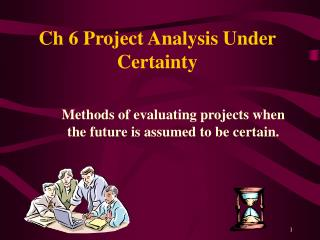DownloadDownload PresentationCh 6 Project Analysis Under Certainty

# Ch 6 Project Analysis Under Certainty

Télécharger la présentation## Ch 6 Project Analysis Under Certainty

- - - - - - - - - - - - - - - - - - - - - - - - - - - E N D - - - - - - - - - - - - - - - - - - - - - - - - - - -
##### Presentation Transcript

1. Ch 6 Project Analysis Under Certainty Methods of evaluating projects when the future is assumed to be certain.

2. Introduction The ideal investment decision making technique is Net Present Value. N P V measures the equivalent present wealth contributed by the investment. NPV is given in NPV -- relates directly to the firm’s goal of wealth maximization -- employs the time value of money -- can be used in all types of investments -- can be adjusted to incorporate risk.

3. Other Project Evaluation Techniques: Slide I Discounted Cash Flow Techniques Internal Rate of Return – calculates the discount rate that gives the project an NPV of \$0. If the IRR is greater than the required rate, the project is accepted. IRR is given as % pa.

4. Other Project Evaluation Techniques: Slide II Modified Internal Rate of Return – calculates the discount rate that gives the project an NPV of \$0, when future cash flows can be re-invested at the Re-Investment Rate, a rate different from the IRR. If the MIRR is greater that the required rate, the project is accepted. MIRR is given as % pa.

5. Other Project Evaluation Techniques: Slide III Non-Discounted Cash Flow Techniques Accounting Rate of Return- measures the ratio of annual average accounting income to an asset base value. ARR is given as % pa. = % pa. Payback Period – measures the length of time required to retrieve the initial cash outlay. PB is given as number of years.

6. Selection of Techniques: Slide I NPV is the technique of choice; it satisfies the requirements of: the firm’s goal, the time value of money, and the absolute measure of investment. IRRis useful in a single asset case, where the cash flow pattern is an outflow followed by all positive inflows. In other situations the IRR may not rank mutually exclusive assets properly, or may have zero or many solutions.

7. Selection of Techniques: Slide II • MIRR is useful in the same situations as the IRR, but requires the extra prediction of a re-investment rate. • ARR allows many valuations of the asset base, does not account for the time value of money, and does not relate to the firm’s goal. It is not a recommended method. • PB does not allow for the time value of money, and does not relate to the firm’s goal. It is not a recommended method.

8. The Notion of Certainty • Certainty allows demonstration and evaluation of the capital budgeting techniques, whilst avoiding the complexities involved with risk. • Certainty requires forecasting, but forecasts which are certain. • Certainty is useful for calculation practice. • Risk is added as an adaption of an evaluation model developed under certainty.

9. Investment Cash Flow Timing End Of Year cash flow timing is assumed. Capital Flows 0 1 2 3 4 5 Initial Later Terminal Outlay. Outlay Flow Operating Flows 0 1 2 3 4 5 Operating Flows.

10. Net annual cash flows are forecast for each year of the project. The NPV Process: Slide I EOY 0 -900 EOY 1 300 EOY 2 380 EOY 3 600 • The discount rate is then applied. -900

11. The NPV Process: Slide II -\$900 \$283.01 \$338.19 \$503.77 The NPV is calculated. NPV = \$ 224.97 Positive: the project is acceptable.

12. Other NPV Applications: Slide I Asset Retirement: Asset Replacement:

13. Other NPV Applications:Slide II • Optimum cycle length within a replacement chain. At EOY: 3691215 OR ? 4 8 12 16

14. Where projects have different lives. Other NPV Applications: Slide III Correct ranking of mutually exclusive projects. Where projects have different outlays.

15. Net Present Value THE model to use in all investment evaluations. Other criteria, such as IRR, MIRR, ARR, and Payback may be used as complementary measures. The End